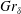Home

# Stability of a temporally evolving natural convection boundary layer on an isothermal wall

## Abstract

The stability properties of a natural convection boundary layer adjacent to an isothermally heated vertical wall, with Prandtl number 0.71, are numerically investigated in the configuration of a temporally evolving parallel flow. The instantaneous linear stability of the flow is first investigated by solving the eigenvalue problem with a quasi-steady assumption, whereby the unsteady base flow is frozen in time. Temporal responses of the discrete perturbation modes are numerically obtained by solving the two-dimensional linearized disturbance equations using a ‘frozen’ base flow as an initial-value problem at various$Gr_{\unicode[STIX]{x1D6FF}}$ , where$Gr_{\unicode[STIX]{x1D6FF}}$ is the Grashof number based on the velocity integral boundary layer thickness$\unicode[STIX]{x1D6FF}$ . The resultant amplification rates of the discrete modes are compared with the quasi-steady eigenvalue analysis, and both two-dimensional and three-dimensional direct numerical simulations (DNS) of the temporally evolving flow. The amplification rate predicted by the linear theory compares well with the result of direct numerical simulation up to a transition point. The extent of the linear regime where the perturbations linearly interact with the base flow is thus identified. The value of the transition$Gr_{\unicode[STIX]{x1D6FF}}$ , according to the three-dimensional DNS results, is dependent on the initial perturbation amplitude. Beyond the transition point, the DNS results diverge from the linear stability predictions as nonlinear mechanisms become important.

## References

Hide All
MathJax
MathJax is a JavaScript display engine for mathematics. For more information see http://www.mathjax.org.

# Stability of a temporally evolving natural convection boundary layer on an isothermal wall

## Metrics

### Full text viewsFull text views reflects the number of PDF downloads, PDFs sent to Google Drive, Dropbox and Kindle and HTML full text views.

Total number of HTML views: 0
Total number of PDF views: 0 *Loading metrics...

### Abstract viewsAbstract views reflect the number of visits to the article landing page.

Total abstract views: 0 *Loading metrics...

* Views captured on Cambridge Core between <date>. This data will be updated every 24 hours.

Usage data cannot currently be displayed